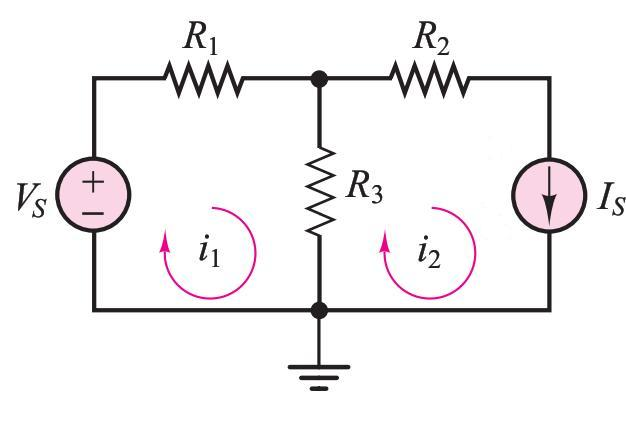# Mesh analysis, 2 meshes 1 known current source

mishima

## Homework Statement

Find i1 in the following figure, given: Vs=10V, I=1A, R1=5, R2=2, R3=4KVL

## The Attempt at a Solution

Applying KVL to mesh 1:
Vs-i1R1-(i1-i2)R3=0
and i2=I=1A
So,
i1=(Vs+i2R3)/(R1+R3)=(10+1*4)/(5+4)=14/9=1.56 A

But book gives answer as 1.71 A.